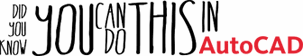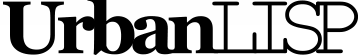# Relative Divide Increasing

With 'Relative Divide Increasing' you can place blocks on linear elements with an increasing scale. You can define a start value and an end value or you can define a start value and an increment. If you are using an increment every next block on the linear entity will be that much bigger. So for instance if your start scale is 1.0 and your increment is 0.5 the blocks will be scaled 1.0 1.5 2.0 2.5 3.0 and so on. When you define an end scale and there are six blocks placed the scales will be 1.0 1.2 1.4 1.6 1.8 and 2.0. If there are only five blocks the scales will be 1.00 1.25 1.50 1.75 and 2.00. The amount of blocks that are placed depends on the distance you specify instead of a number of segments. The command will place as much blocks as possible on the linear entities but will alway keep the distance you specify between the blocks. Or you can use the distance as a maximum. Either way the blocks are spread equally along the linear entities but you know there's a certain amount of distance between the blocks.

## Command summery

Entry:
RDN
Applies to:
polylines, circles, ellipses, splines, lines, arcs
Effect:
Place blocks on selected linear entities with an increasing scale with the divide method based on a distance
Tags:
distance, minimum, maximum, align, group, scale,
Categories:
Blocks, inserters
Suitability: